# Quadratic Equation for IBPS , SBI , RBI | Quantitative Aptitude | Quiz-1

## Quadratic Equation for IBPS , SBI , RBI:

Quadratic equation Quiz for Bank pre exam : Quadratic equation is an important chapter of quantitative aptitude in competitive exams such as IBPS, SBI and RBI PO & Clerk. Solving more and more Quadratic equation Questions will give you a good command on this topic. So, here we are giving you Quadratic equation Questions and answers for IBPS, SBI and RBI PO & Clerk exam. This Quadratic equation Quiz contains questions based on the latest exam pattern of IBPS, SBI and RBI PO & Clerk exam. Here, answers and solution are also provided in this Quadratic equation quiz. Attempt this Quadratic equation Quiz and practice from it to master this topic.

Quadratic Equation Quiz to improve your Quantitative Aptitude for SBI Po & SBI clerk exam IBPS PO Reasoning , IBPS Clerk Reasoning , IBPS RRB Reasoning, LIC AAO ,LIC Assistant  and other competitive exam

Directions (1 – 5): In each of these questions, two equations (I) and (II) are given. You have to solve both the equations and give answer.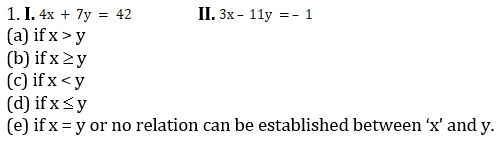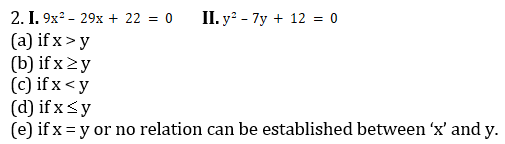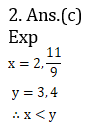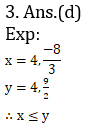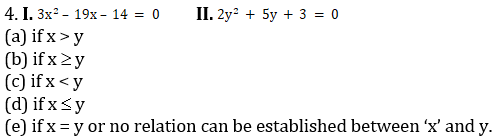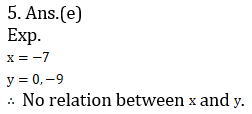Directions (6-10): Two equations (I) and (II) are given in each question. On the basis of these equations, you have to decide the relation between x and y and give answer.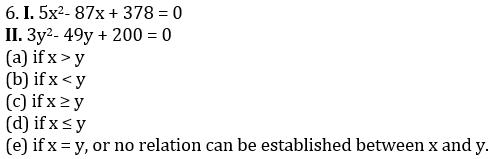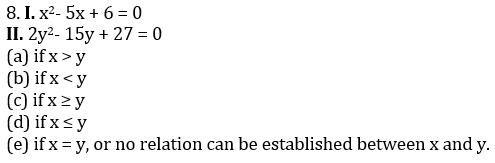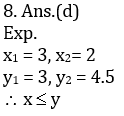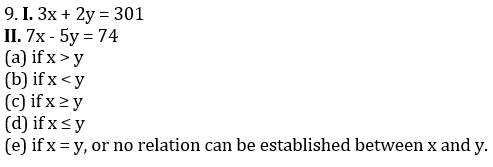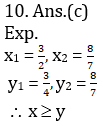### Online Mock Test Available on App as well as Web:### How to Access on App:-

1. Go to Playstore search Ambitious Baba or Click here to Install App

### You Can Read This Also:

ambitiousbaba.com need your support to Grow

I challenge you will get Best Content in Our PDFs with Detail solutions and Latest Pattern

Memory Based Puzzle E-book | 2016-19 Exams Covered

Get PDF here

Caselet Data Interpretation 200 Questions

Get PDF here
Puzzle & Seating Arrangement E-Book for BANK PO MAINS (Vol-1)

Get PDF here

### ARITHMETIC DATA INTERPRETATION 2020 E-book

Get PDF here
The Banking Awareness 500 MCQs E-book| Bilingual (Hindi + English)

Get PDF here

High Level DATA INTERPRETATION Practice E-BOOK

Get PDF her